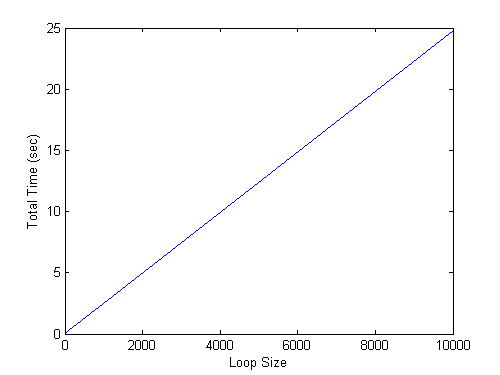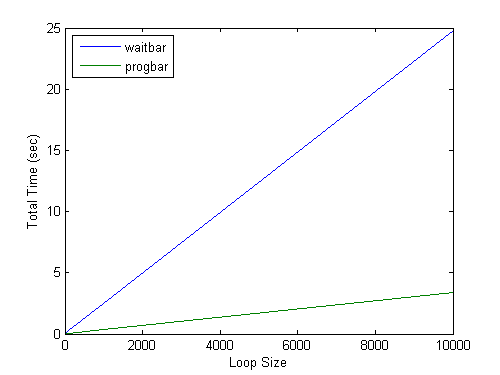# progbar

Bob's pick this week is progbar by Ben Mitch.

Do your MATLAB programs take a long time to run? Maybe you have a large simulation that loops over many iterations. It's nice to monitor progress. You can do that with waitbar.

h = waitbar(0,'Please wait...');
for step = 1:1000
% computations take place here
waitbar(step/1000)
drawnow
end
close(h)

That works well for coarse loops with few iterations and considerable time spent during each. The instrumentation itself does add some overhead, however. As the number of iterations increases, so does the cumulative time.

loopSizes = [1 10 100 1000 10e3];
waitbarTimes = zeros(size(loopSizes));
for n=1:numel(loopSizes)
myBar = waitbar(0,num2str(loopSizes(n)));
myClock = tic;
for k=1:loopSizes(n)
waitbar(k/loopSizes(n),myBar)
drawnow
end %loop simulation
waitbarTimes(n) = toc(myClock);
close(myBar)
end %vary problem size
plot(loopSizes,waitbarTimes)
xlabel 'Loop Size'
ylabel 'Total Time (sec)'Since a loop of ten thousand iterations added that many seconds of overhead, how many minutes would a loop of one million add?

100*waitbarTimes(end)/60 % 100X bigger loop (sec->min)
ans =
41.332


Wow! If your whole simulation only takes a few minutes total to run then you probably don't want to bog it down that much by over instrumenting it.

myBar = waitbar(0,'Big loop with coarse updates');
myClock = tic;
for step=1:1e4
% computations take place here
if mod(step,10)==0 %every 10-th iteration
waitbar(step/1e4,myBar)
drawnow
end
end
toc(myClock)
close(myBar)
Elapsed time is 2.613291 seconds.


Of course now your scientific task involves some artistry to chose the right number of iterations to skip each time. However, suppose the amount of time varies widely between each iteration. This approach falls apart in that case.

What I like about progbar is that it features an update "period" setting you can control. The default is 0.1 seconds. So it will not update more than 10 times per second. Otherwise, it works (not exactly but) a lot like waitbar.

myBar = progbar;
myClock = tic;
for step=1:1e4
% computations take place here
progbar(myBar,100*step/1e4)
drawnow
end
toc(myClock)
close(myBar)
Elapsed time is 3.375511 seconds.


If we vary the loop size again we can see where progbar begins to shine.

progbarTimes = zeros(size(loopSizes));
for n=1:numel(loopSizes)
myBar = progbar;
myClock = tic;
for k=1:loopSizes(n)
progbar(myBar,100*k/loopSizes(n))
drawnow
end %loop simulation
progbarTimes(n) = toc(myClock);
close(myBar)
end %vary problem size
plot(loopSizes,[waitbarTimes' progbarTimes'])
xlabel 'Loop Size'
ylabel 'Total Time (sec)'
legend waitbar progbar Location Northwest